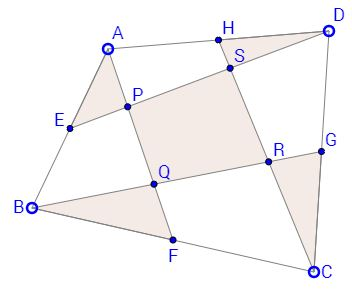Carpets in a Quadrilateral II

Created with GeoGebra

$E,\,$ $F,\,$ $G,\,$ $H\,$ are the midpoints of sides $AB,\,$ $BC,\,$ $CD,\,$ $DA\,$ of a convex quadrilateral $ABCD.\,$ $P\,$ is the intersection of $AF, DE,\,$ $Q\,$ the intersection of $AF, BG,\,$ $R\,$ the intersection of $BG, CH,\,$ and $S\,$ the intersection of $CH, DE.\,$ Then

$[\Delta AEP] + [\Delta BFQ] + [\Delta CGR] + [\Delta DHS] = [PQRS],$

where $[X]\,$ denotes the area of shape $X.$

ExplanationThis problem was suggested by Bui Quang Tuan. Although simple, I do not have a recollection of seeing it anywhere else. (P.S. Years later I came across this problem in the highly recommended Mathematical Olympiad Treasures by T. Andreescu, B. Enescu (Birkhäuser, 2004).)

$E,\,$ $F,\,$ $G,\,$ $H\,$ are the midpoints of sides $AB,\,$ $BC,\,$ $CD,\,$ $DA\,$ of a convex quadrilateral $ABCD.\,$ $P\,$ is the intersection of $AF, DE,\,$ $Q\,$ the intersection of $AF, BG,\,$ $R\,$ the intersection of $BG, CH,\,$ and $S\,$ the intersection of $CH, DE.\,$ Then$[\Delta AEP] + [\Delta BFQ] + [\Delta CGR] + [\Delta DHS] = [PQRS],$

The solution is a direct consequence of the Carpet's Theorem.

We split the quadrilateral in two ways

$S_{1} \cup S_{2} = T_{1} \cup T_{2},$

where $S_{1}\,$ is the quadrilateral $AFCH\,$ and $S_{2}\,$ is the union of the remaining two triangles. Similarly, $T_{1}\,$ is the quadrilateral $BGDE\,$ and $T_{2}\,$ its complement in the quadrilateral. Note that the area of each of the four regions is half that of the quadrilateral. For example,

$[\Delta ADE] = [\Delta BDE]\,$ and
$[\Delta BCG] = [\Delta BDG].$

Adding the two gives

$[\Delta ADE] + [\Delta BCG] = [\Delta BDE] + [\Delta BDG] = [BGDE].$

By the Carpet's Theorem,

$[S_{1} \cap T_{1}] = [S_{2} \cap T_{2}].$

But $S_{1} \cap T_{1}\,$ is the quadrilateral $PQRS,\,$ whereas $S_{2}\,$ \cap $T_{2}\,$ is the union of triangles $AEP,\,$ $BFQ,\,$ $CGR,\,$ and $DHS,\,$ as required.Remark

In fact more is true. Mariano Perez de la Cruz has observed that the problem is of affine nature. The midpoints are preserved by affine transformations (homothety, reflection, translation, shearing) and, more generally, so are the ratio of any two lengths and any two areas. Any convex quadrilateral can be obtained from a square by an affine transformation. In a square, joining the vertices to midpoints of the sides leaves off a square with $\displaystyle \frac{1}{5}\,$ of the area of the original square. This is then true for the two quadrilaterals $ABCD\,$ and $PQRS.$Carpets Theorem